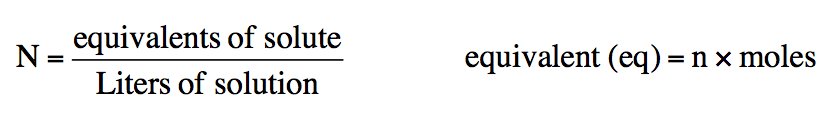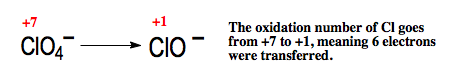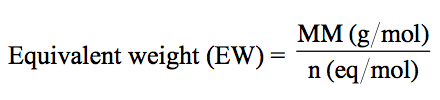Ch.4 - Chemical Quantities & Aqueous ReactionsWorksheetSee all chapters
 Ch.1 - Intro to General Chemistry 2hrs & 53mins 0% complete Worksheet Ch.2 - Atoms & Elements 2hrs & 49mins 0% complete Worksheet Ch.3 - Chemical Reactions 3hrs & 25mins 0% complete Worksheet BONUS: Lab Techniques and Procedures 1hr & 38mins 0% complete Worksheet BONUS: Mathematical Operations and Functions 47mins 0% complete Worksheet Ch.4 - Chemical Quantities & Aqueous Reactions 3hrs & 55mins 0% complete Worksheet Ch.5 - Gases 3hrs & 47mins 0% complete Worksheet Ch.6 - Thermochemistry 2hrs & 28mins 0% complete Worksheet Ch.7 - Quantum Mechanics 2hrs & 35mins 0% complete Worksheet Ch.8 - Periodic Properties of the Elements 1hr & 57mins 0% complete Worksheet Ch.9 - Bonding & Molecular Structure 2hrs & 5mins 0% complete Worksheet Ch.10 - Molecular Shapes & Valence Bond Theory 1hr & 31mins 0% complete Worksheet Ch.11 - Liquids, Solids & Intermolecular Forces 3hrs & 40mins 0% complete Worksheet Ch.12 - Solutions 2hrs & 17mins 0% complete Worksheet Ch.13 - Chemical Kinetics 2hrs & 22mins 0% complete Worksheet Ch.14 - Chemical Equilibrium 2hrs & 26mins 0% complete Worksheet Ch.15 - Acid and Base Equilibrium 4hrs & 42mins 0% complete Worksheet Ch.16 - Aqueous Equilibrium 3hrs & 48mins 0% complete Worksheet Ch. 17 - Chemical Thermodynamics 1hr & 44mins 0% complete Worksheet Ch.18 - Electrochemistry 2hrs & 58mins 0% complete Worksheet Ch.19 - Nuclear Chemistry 1hr & 33mins 0% complete Worksheet Ch.20 - Organic Chemistry 3hrs 0% complete Worksheet Ch.22 - Chemistry of the Nonmetals 2hrs & 1min 0% complete Worksheet Ch.23 - Transition Metals and Coordination Compounds 1hr & 54mins 0% complete Worksheet

# Normality & Equivalent Weight

See all sections

Normality represents the number of equivalents per liter of solution.

###### Understanding Normality

Concept #1: Understanding NormalityAn equivalent is the mass of a compound that can either donate an H(Acids), accept an H+ (Bases) or transfer an electron (in Redox Reactions).

Example #1: Determine the number of equivalents for each of the acids given.

For an acid the variable n is based on the number of H+ ions it can donate.

Example #2: Determine the number of equivalents for the following base.

For a base the variable n is based on the number of hydroxide, OH, ions present.

Example #3: Based on the given redox reaction determine the value for n.For a redox reaction the number for n is based on the number of electrons transferred.

###### Calculations with Normality

Example #4: What is the normality of a solution made by dissolving 325.1 g HNO3 in enough water to create a 750.0 mL solution?

Example #5: Determine the equivalent weight of the following compounds.

The equivalent weight of a compound is equal to its molecular mass divided by the variable nExample #6: What volume, in mL, of 50.0 g H2SO4 is needed to create a 0.300 N H2SO4 solution?

Example #7: If a concentrated 3.25 M H3PO4 solution possesses a density of 1.350 g/mL, what is its normality?